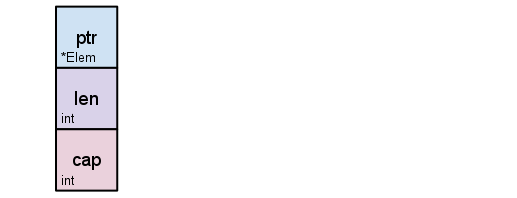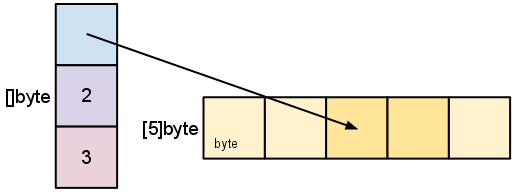## Golang 中的slice

PHP写久了，对各种数据类型就不太在意了。一开始对Golang中slice类型的理解就是一个简单的array，今天在刷leetcode的时候碰到一个很有意思的题目Remove Duplicates from Sorted Array 题目描述如下：

Given a sorted array, remove the duplicates in place such that each element appear only once and return the new length.

Do not allocate extra space for another array, you must do this in place with constant memory.

For example,
Given input array nums = [1,1,2],

Your function should return length = 2, with the first two elements of nums being 1 and 2 respectively. It doesn't matter what you leave beyond the new length.s=s[2:4] 对切片重新切片d := []byte{'r', 'o', 'a', 'd'}
e := d[2:]
// e []byte{'a', 'd'}
e = 'm'
// e
[]byte{'a', 'm'}
// d == []byte{'r', 'o', 'a', 'm'}

func deleteNode(nums []int,index int) []int{
nums=append(nums[:index],nums[index+1]...)
return nums
}